## EXERCISE 3.1 PAGE NO: 3.4

1. Which of the following numbers are perfect squares?
(i) 484
(ii) 625
(iii) 576
(iv) 941
(v) 961
(vi) 2500
Solution:
(i) 484
First find the prime factors for 484
484 = 2×2×11×11
By grouping the prime factors in equal pairs we get,
= (2×2) × (11×11)
By observation, none of the prime factors are left out.
∴ 484 is a perfect square.
(ii) 625
First find the prime factors for 625
625 = 5×5×5×5
By grouping the prime factors in equal pairs we get,
= (5×5) × (5×5)
By observation, none of the prime factors are left out.
∴ 625 is a perfect square.
(iii) 576
First find the prime factors for 576
576 = 2×2×2×2×2×2×3×3
By grouping the prime factors in equal pairs we get,
= (2×2) × (2×2) × (2×2) × (3×3)
By observation, none of the prime factors are left out.
∴ 576 is a perfect square.
(iv) 941
First find the prime factors for 941
941 = 941 × 1
We know that 941 itself is a prime factor.
∴ 941 is not a perfect square.
(v) 961
First find the prime factors for 961
961 = 31×31
By grouping the prime factors in equal pairs we get,
= (31×31)
By observation, none of the prime factors are left out.
∴ 961 is a perfect square.
(vi) 2500
First find the prime factors for 2500
2500 = 2×2×5×5×5×5
By grouping the prime factors in equal pairs we get,
= (2×2) × (5×5) × (5×5)
By observation, none of the prime factors are left out.
∴ 2500 is a perfect square.
2. Show that each of the following numbers is a perfect square. Also find the number whose square is the given number in each case:
(i) 1156
(ii) 2025
(iii) 14641
(iv) 4761
Solution:
(i) 1156
First find the prime factors for 1156
1156 = 2×2×17×17
By grouping the prime factors in equal pairs we get,
= (2×2) × (17×17)
By observation, none of the prime factors are left out.
∴ 1156 is a perfect square.
To find the square of the given number
1156 = (2×17) × (2×17)
= 34 × 34
= (34)2
∴ 1156 is a square of 34.
(ii) 2025
First find the prime factors for 2025
2025 = 3×3×3×3×5×5
By grouping the prime factors in equal pairs we get,
= (3×3) × (3×3) × (5×5)
By observation, none of the prime factors are left out.
∴ 2025 is a perfect square.
To find the square of the given number
2025 = (3×3×5) × (3×3×5)
= 45 × 45
= (45)2
∴ 2025 is a square of 45.
(iii) 14641
First find the prime factors for 14641
14641 = 11×11×11×11
By grouping the prime factors in equal pairs we get,
= (11×11) × (11×11)
By observation, none of the prime factors are left out.
∴ 14641 is a perfect square.
To find the square of the given number
14641 = (11×11) × (11×11)
= 121 × 121
= (121)2
∴ 14641 is a square of 121.
(iv) 4761
First find the prime factors for 4761
4761 = 3×3×23×23
By grouping the prime factors in equal pairs we get,
= (3×3) × (23×23)
By observation, none of the prime factors are left out.
∴ 4761 is a perfect square.
To find the square of the given number
4761 = (3×23) × (3×23)
= 69 × 69
= (69)2
∴ 4761 is a square of 69.
3. Find the smallest number by which the given number must be multiplied so that the product is a perfect square:
(i) 23805
(ii) 12150
(iii) 7688
Solution:
(i) 23805
First find the prime factors for 23805
23805 = 3×3×23×23×5
By grouping the prime factors in equal pairs we get,
= (3×3) × (23×23) × 5
By observation, prime factor 5 is left out.
So, multiply by 5 we get,
23805 × 5 = (3×3) × (23×23) × (5×5)
= (3×5×23) × (3×5×23)
= 345 × 345
= (345)2
∴ Product is the square of 345.
(ii) 12150
First find the prime factors for 12150
12150 = 2×2×2×2×3×3×5×5×2
By grouping the prime factors in equal pairs we get,
= (2×2) × (2×2) × (3×3) × (5×5) × 2
By observation, prime factor 2 is left out.
So, multiply by 2 we get,
12150 × 2 = (2×2) × (2×2) × (3×3) × (5×5) × (2×2)
= (2×2×3×5×2) × (2×2×3×5×2)
= 120 × 120
= (120)2
∴ Product is the square of 120.
(iii) 7688
First find the prime factors for 7688
7688 = 2×2×31×31×2
By grouping the prime factors in equal pairs we get,
= (2×2) × (31×31) × 2
By observation, prime factor 2 is left out.
So, multiply by 2 we get,
7688 × 2 = (2×2) × (31×31)× (2×2)
= (2×31×2) × (2×31×2)
= 124 × 124
= (124)2
∴ Product is the square of 124.
4. Find the smallest number by which the given number must be divided so that the resulting number is a perfect square:
(i) 12283
(ii) 1800
(iii) 2904
Solution:
(i) 12283
First find the prime factors for 12283
12283 = 3×3×3×23×23
By grouping the prime factors in equal pairs we get,
= (3×3) × (23×23) × 3
By observation, prime factor 3 is left out.
So, divide by 3 to eliminate 3 we get,
12283/3 = (3×3) × (23×23)
= (3×23) × (3×23)
= 69 × 69
= (69)2
∴ Resultant is the square of 69.
(ii) 1800
First find the prime factors for 1800
1800 = 2×2×5×5×3×3×2
By grouping the prime factors in equal pairs we get,
= (2×2) × (5×5) × (3×3) × 2
By observation, prime factor 2 is left out.
So, divide by 2 to eliminate 2 we get,
1800/2 = (2×2) × (5×5) × (3×3)
= (2×5×3) × (2×5×3)
= 30 × 30
= (30)2
∴ Resultant is the square of 30.
(iii) 2904
First find the prime factors for 2904
2904 = 2×2×11×11×2×3
By grouping the prime factors in equal pairs we get,
= (2×2) × (11×11) × 2 × 3
By observation, prime factor 2 and 3 are left out.
So, divide by 6 to eliminate 2 and 3 we get,
2904/6 = (2×2) × (11×11)
= (2×11) × (2×11)
= 22 × 22
= (22)2
∴ Resultant is the square of 22.
5. Which of the following numbers are perfect squares?
11, 12, 16, 32, 36, 50, 64, 79, 81, 111, 121
Solution:
11 it is a prime number by itself.
So it is not a perfect square.
12 is not a perfect square.
16= (4)2
16 is a perfect square.
32 is not a perfect square.
36= (6)2
36 is a perfect square.
50 is not a perfect square.
64= (8)2
64 is a perfect square.
79 it is a prime number.
So it is not a perfect square.
81= (9)2
81 is a perfect square.
111 it is a prime number.
So it is not a perfect square.
121= (11)2
121 is a perfect square.
6. Using prime factorization method, find which of the following numbers are perfect squares?
189, 225, 2048, 343, 441, 2961, 11025, 3549
Solution:
189 prime factors are
189 = 32×3×7
Since it does not have equal pair of factors 189 is not a perfect square.
225 prime factors are
225 = (5×5) × (3×3)
Since 225 has equal pair of factors. ∴ It is a perfect square.
2048 prime factors are
2048 = (2×2) × (2×2) × (2×2) × (2×2) × (2×2) × 2
Since it does not have equal pair of factors 2048 is not a perfect square.
343 prime factors are
343 = (7×7) × 7
Since it does not have equal pair of factors 2048 is not a perfect square.
441 prime factors are
441 = (7×7) × (3×3)
Since 441 has equal pair of factors. ∴ It is a perfect square.
2961 prime factors are
2961 = (3×3) × (3×3) × (3×3) × (2×2)
Since 2961 has equal pair of factors. ∴ It is a perfect square.
11025 prime factors are
11025 = (3×3) × (5×5) × (7×7)
Since 11025 has equal pair of factors. ∴ It is a perfect square.
3549 prime factors are
3549 = (13×13) × 3 × 7
Since it does not have equal pair of factors 3549 is not a perfect square.
7. By what number should each of the following numbers by multiplied to get a perfect square in each case? Also find the number whose square is the new number.
(i) 8820
(ii) 3675
(iii) 605
(iv) 2880
(v) 4056
(vi) 3468
(vii) 7776
Solution:
(i) 8820
First find the prime factors for 8820
8820 = 2×2×3×3×7×7×5
By grouping the prime factors in equal pairs we get,
= (2×2) × (3×3) × (7×7) × 5
By observation, prime factor 5 is left out.
So, multiply by 5 we get,
8820 × 5 = (2×2) × (3×3) × (7×7) × (5×5)
= (2×3×7×5) × (2×3×7×5)
= 210 × 210
= (210)2
∴ Product is the square of 210.
(ii) 3675
First find the prime factors for 3675
3675 = 5×5×7×7×3
By grouping the prime factors in equal pairs we get,
= (5×5) × (7×7) × 3
By observation, prime factor 3 is left out.
So, multiply by 3 we get,
3675 × 3 = (5×5) × (7×7) × (3×3)
= (5×7×3) × (5×7×3)
= 105 × 105
= (105)2
∴ Product is the square of 105.
(iii) 605
First find the prime factors for 605
605 = 5×11×11
By grouping the prime factors in equal pairs we get,
= (11×11) × 5
By observation, prime factor 5 is left out.
So, multiply by 5 we get,
605 × 5 = (11×11) × (5×5)
= (11×5) × (11×5)
= 55 × 55
= (55)2
∴ Product is the square of 55.
(iv) 2880
First find the prime factors for 2880
2880 = 5×3×3×2×2×2×2×2×2
By grouping the prime factors in equal pairs we get,
= (3×3) × (2×2) × (2×2) × (2×2) × 5
By observation, prime factor 5 is left out.
So, multiply by 5 we get,
2880 × 5 = (3×3) × (2×2) × (2×2) × (2×2) × (5×5)
= (3×2×2×2×5) × (3×2×2×2×5)
= 120 × 120
= (120)2
∴ Product is the square of 120.
(v) 4056
First find the prime factors for 4056
4056 = 2×2×13×13×2×3
By grouping the prime factors in equal pairs we get,
= (2×2) × (13×13) × 2 × 3
By observation, prime factors 2 and 3 are left out.
So, multiply by 6 we get,
4056 × 6 = (2×2) × (13×13) × (2×2) × (3×3)
= (2×13×2×3) × (2×13×2×3)
= 156 × 156
= (156)2
∴ Product is the square of 156.
(vi) 3468
First find the prime factors for 3468
3468 = 2×2×17×17×3
By grouping the prime factors in equal pairs we get,
= (2×2) × (17×17) × 3
By observation, prime factor 3 is left out.
So, multiply by 3 we get,
3468 × 3 = (2×2) × (17×17) × (3×3)
= (2×17×3) × (2×17×3)
= 102 × 102
= (102)2
∴ Product is the square of 102.
(vii) 7776
First find the prime factors for 7776
7776 = 2×2×2×2×3×3×3×3×2×3
By grouping the prime factors in equal pairs we get,
= (2×2) × (2×2) × (3×3) × (3×3) × 2 × 3
By observation, prime factors 2 and 3 are left out.
So, multiply by 6 we get,
7776 × 6 = (2×2) × (2×2) × (3×3) × (3×3) × (2×2) × (3×3)
= (2×2×3×3×2×3) × (2×2×3×3×2×3)
= 216 × 216
= (216)2
∴ Product is the square of 216.
8. By What numbers should each of the following be divided to get a perfect square in each case? Also, find the number whose square is the new number.
(i) 16562
(ii) 3698
(iii) 5103
(iv) 3174
(v) 1575
Solution:
(i) 16562
First find the prime factors for 16562
16562 = 7×7×13×13×2
By grouping the prime factors in equal pairs we get,
= (7×7) × (13×13) × 2
By observation, prime factor 2 is left out.
So, divide by 2 to eliminate 2 we get,
16562/2 = (7×7) × (13×13)
= (7×13) × (7×13)
= 91 × 91
= (91)2
∴ Resultant is the square of 91.
(ii) 3698
First find the prime factors for 3698
3698 = 2×43×43
By grouping the prime factors in equal pairs we get,
= (43×43) × 2
By observation, prime factor 2 is left out.
So, divide by 2 to eliminate 2 we get,
3698/2 = (43×43)
= (43)2
∴ Resultant is the square of 43.
(iii) 5103
First find the prime factors for 5103
5103 = 3×3×3×3×3×3×7
By grouping the prime factors in equal pairs we get,
= (3×3) × (3×3) × (3×3) × 7
By observation, prime factor 7 is left out.
So, divide by 7 to eliminate 7 we get,
5103/7 = (3×3) × (3×3) × (3×3)
= (3×3×3) × (3×3×3)
= 27 × 27
= (27)2
∴ Resultant is the square of 27.
(iv) 3174
First find the prime factors for 3174
3174 = 2×3×23×23
By grouping the prime factors in equal pairs we get,
= (23×23) × 2 × 3
By observation, prime factor 2 and 3 are left out.
So, divide by 6 to eliminate 2 and 3 we get,
3174/6 = (23×23)
= (23)2
∴ Resultant is the square of 23.
(v) 1575
First find the prime factors for 1575
1575 = 3×3×5×5×7
By grouping the prime factors in equal pairs we get,
= (3×3) × (5×5) × 7
By observation, prime factor 7 is left out.
So, divide by 7 to eliminate 7 we get,
1575/7 = (3×3) × (5×5)
= (3×5) × (3×5)
= 15 × 15
= (15)2
∴ Resultant is the square of 15.
9. Find the greatest number of two digits which is a perfect square.
Solution:
We know that the two digit greatest number is 99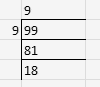∴ Greatest two digit perfect square number is 99-18 = 81
10. Find the least number of three digits which is perfect square.
Solution:
We know that the three digit greatest number is 100
To find the square root of 100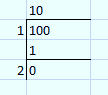∴ the least number of three digits which is a perfect square is 100 itself.
11. Find the smallest number by which 4851 must be multiplied so that the product becomes a perfect square.
Solution:
First find the prime factors for 4851
4851 = 3×3×7×7×11
By grouping the prime factors in equal pairs we get,
= (3×3) × (7×7) × 11
∴ The smallest number by which 4851 must be multiplied so that the product becomes a perfect square is 11.
12. Find the smallest number by which 28812 must be divided so that the quotient becomes a perfect square.
Solution:
First find the prime factors for 28812
28812 = 2×2×3×3×3×17×17
By grouping the prime factors in equal pairs we get,
= (2×2) × (3×3) × (17×17) × 3
∴ The smallest number by which 28812 must be divided so that the quotient becomes a perfect square is 3.
13. Find the smallest number by which 1152 must be divided so that it becomes a perfect square. Also find the number whose square is the resulting number.
Solution:
First find the prime factors for 1152
28812 = 2×2×2×2×2×2×2×3×3
By grouping the prime factors in equal pairs we get,
= (2×2) × (2×2) × (2×2) × (3×3) × 3
∴ The smallest number by which 1152 must be divided so that the quotient becomes a perfect square is 2.
The number after division, 1152/2 = 576
prime factors for 576 = 2×2×2×2×2×2×3×3
By grouping the prime factors in equal pairs we get,
= (2×2) × (2×2) × (2×2) × (3×3)
= 26 × 32
= 242
∴ The resulting number is the square of 24.

## EXERCISE 3.2 PAGE NO: 3.18

1. The following numbers are not perfect squares. Give reason.
(i) 1547
(ii) 45743
(iii)8948
(iv) 333333
Solution:
The numbers ending with 2, 3, 7 or 8 is not a perfect square.
So, (i) 1547
(ii) 45743
(iii)
8948
(iv) 333333
Are not perfect squares.
2. Show that the following numbers are not, perfect squares:
(i) 9327
(ii) 4058
(iii)22453
(iv) 743522
Solution:
The numbers ending with 2, 3, 7 or 8 is not a perfect square.
So, (i) 9327
(ii) 4058
(iii) 22453
(iv) 743522
Are not perfect squares.
3. The square of which of the following numbers would be an old number?
(i) 731
(ii) 3456
(iii)5559
(iv) 42008
Solution:
We know that square of an even number is even number.
Square of an odd number is odd number.
(i) 731
Since 731 is an odd number, the square of the given number is also odd.
(ii) 3456
Since 3456 is an even number, the square of the given number is also even.
(iii) 5559
Since 5559 is an odd number, the square of the given number is also odd.
(iv) 42008
Since 42008 is an even number, the square of the given number is also even.
4. What will be the unit’s digit of the squares of the following numbers?
(i) 52
(ii) 977
(iii) 4583
(iv) 78367
(v) 52698
(vi) 99880
(vii) 12796
(viii) 55555
(ix) 53924
Solution:
(i) 52
Unit digit of (52)2 = (22) = 4
(ii) 977
Unit digit of (977)2 = (72) = 49 = 9
(iii) 4583
Unit digit of (4583)2 = (32) = 9
(iv) 78367
Unit digit of (78367)2 = (72) = 49 = 9
(v) 52698
Unit digit of (52698)2 = (82) = 64 = 4
(vi) 99880
Unit digit of (99880)2 = (02) = 0
(vii) 12796
Unit digit of (12796)2 = (62) = 36 = 6
(viii) 55555
Unit digit of (55555)2 = (52) = 25 = 5
(ix) 53924
Unit digit of (53924)2 = (42) = 16 = 6
5. Observe the following pattern
1+3 = 22
1+3+5 = 32
1+3+5+7 = 42
And write the value of 1+3+5+7+9+……… up to n terms.
Solution:
We know that the pattern given is the square of the given number on the right hand side is equal to the sum of the given numbers on the left hand side.
∴ The value of 1+3+5+7+9+……… up to n terms = n2 (as there are only n terms).
6. Observe the following pattern
22 -12 = 2 + 1
32 – 22 = 3 + 2
42 – 32 = 4 + 3
52 – 42 = 5 + 4
And find the value of
(i) 1002 -992
(ii)1112 – 1092
(iii) 992 – 962
Solution:
(i) 1002 -992
100 + 99 = 199
(ii) 1112 – 1092
(1112 – 1102) + (1102 – 1092)
(111 + 110) + (100 + 109)
440
(iii) 99 – 96
(992 – 982) + (982 – 972) + (972 – 962)
(99 + 98) + (98 + 97) + (97 + 96)
585
7. Which of the following triplets are Pythagorean?
(i) (8, 15, 17)
(ii) (18, 80, 82)
(iii) (14, 48, 51)
(iv) (10, 24, 26)
(v) (16, 63, 65)
(vi) (12, 35, 38)
Solution:
(i) (8, 15, 17)
LHS = 82 + 152
= 289
RHS = 172
= 289
LHS = RHS
∴ The given triplet is a Pythagorean.
(ii) (18, 80, 82)
LHS = 182 + 802
= 6724
RHS = 822
= 6724
LHS = RHS
∴ The given triplet is a Pythagorean.
(iii) (14, 48, 51)
LHS = 142 + 482
= 2500
RHS = 512
= 2601
LHS ≠ RHS
∴ The given triplet is not a Pythagorean.
(iv) (10, 24, 26)
LHS = 102 + 242
= 676
RHS = 262
= 676
LHS = RHS
∴ The given triplet is a Pythagorean.
(v) (16, 63, 65)
LHS = 162 + 632
= 4225
RHS = 652
= 4225
LHS = RHS
∴ The given triplet is a Pythagorean.
(vi) (12, 35, 38)
LHS = 122 + 352
= 1369
RHS = 382
= 1444
LHS ≠ RHS
∴ The given triplet is not a Pythagorean.
8. Observe the following pattern
(1×2) + (2×3) = (2×3×4)/3
(1×2) + (2×3) + (3×4) = (3×4×5)/3
(1×2) + (2×3) + (3×4) + (4×5) = (4×5×6)/3
And find the value of
(1×2) + (2×3) + (3×4) + (4×5) + (5×6)
Solution:
(1×2) + (2×3) + (3×4) + (4×5) + (5×6) = (5×6×7)/3 = 70
9. Observe the following pattern
1 = 1/2 (1×(1+1))
1+2 = 1/2 (2×(2+1))
1+2+3 = 1/2 (3×(3+1))
1+2+3+4 = 1/2 (4×(4+1))
And find the values of each of the following:
(i) 1+2+3+4+5+…+50
(ii) 31+32+….+50
Solution:
We know that R.H.S = 1/2 [No. of terms in L.H.S × (No. of terms + 1)] (if only when L.H.S starts with 1)
(i) 1+2+3+4+5+…+50 = 1/2 (5×(5+1))
25 × 51 = 1275
(ii) 31+32+….+50 = (1+2+3+4+5+…+50) – (1+2+3+…+30)
1275 – 1/2 (30×(30+1))
1275 – 465
810
10. Observe the following pattern
12 = 1/6 (1×(1+1)×(2×1+1))
12+22 = 1/6 (2×(2+1)×(2×2+1)))
12+22+32 = 1/6 (3×(3+1)×(2×3+1)))
12+22+32+42 = 1/6 (4×(4+1)×(2×4+1)))
And find the values of each of the following:
(i) 12+22+32+42+…+102
(ii) 52+62+72+82+92+102+112+122
Solution:
RHS = 1/6 [(No. of terms in L.H.S) × (No. of terms + 1) × (2 × No. of terms + 1)]
(i) 12+22+32+42+…+102 = 1/6 (10×(10+1)×(2×10+1))
= 1/6 (2310)
= 385
(ii) 52+62+72+82+92+102+112+122 = 12+22+32+…+122 – (12+22+32+42)
1/6 (12×(12+1)×(2×12+1)) – 1/6 (4×(4+1)×(2×4+1))
650-30
620
11. Which of the following numbers are squares of even numbers?
121, 225, 256, 324, 1296, 6561, 5476, 4489, 373758
Solution:
We know that only even numbers be the squares of even numbers.
So, 256, 324, 1296, 5476, 373758 are even numbers, since 373758 is not a perfect square
∴ 256, 324, 1296, 5476 are squares of even numbers.
12. By just examining the units digits, can you tell which of the following cannot be whole squares?
(i) 1026
(ii) 1028
(iii)1024
(iv) 1022
(v) 1023
(vi) 1027
Solution:
We know that numbers ending with 2, 3, 7, 8 cannot be a perfect square.
∴ 1028, 1022, 1023, and 1027 cannot be whole squares.
13. Which of the numbers for which you cannot decide whether they are squares.
Solution:
We know that the natural numbers such as 0, 1, 4, 5, 6 or 9 cannot be decided surely whether they are squares or not.
14. Write five numbers which you cannot decide whether they are square just by looking at the unit’s digit.
Solution:
We know that any natural number ending with 0, 1, 4, 5, 6 or 9 can be or cannot be a square number.
Here are the five examples which you cannot decide whether they are square or not just by looking at the units place:
(i) 2061
The unit digit is 1. So, it may or may not be a square number
(ii) 1069
The unit digit is 9. So, it may or may not be a square number
(iii) 1234
The unit digit is 4. So, it may or may not be a square number
(iv) 56790
The unit digit is 0. So, it may or may not be a square number
(v) 76555
The unit digit is 5. So, it may or may not be a square number
15. Write true (T) or false (F) for the following statements.
(i) The number of digits in a square number is even.
(ii) The square of a prime number is prime.
(iii) The sum of two square numbers is a square number.
(iv) The difference of two square numbers is a square number.
(v) The product of two square numbers is a square number.
(vi) No square number is negative.
(vii) There is no square number between 50 and 60.
(viii) There are fourteen square number up to 200.
Solution:
(i) False, because 169 is a square number with odd digit.
(ii) False, because square of 3(which is prime) is 9(which is not prime).
(iii) False, because sum of 22 and 32 is 13 which is not square number.
(iv) False, because difference of 32 and 22 is 5, which is not square number.
(v) True, because the square of 22 and 32 is 36 which is square of 6
(vi) True, because (-2)2 is 4, which is not negative.
(vii) True, because as there is no square number between them.
(viii) True, because the fourteen numbers up to 200 are: 1, 4, 9, 16, 25, 36, 49, 64, 81, 100, 121, 144, 169, 196.

## EXERCISE 3.3 PAGE NO: 3.32

1. Find the squares of the following numbers using column method. Verify the result by finding the square using the usual multiplication:
(i) 25
(ii) 37
(iii) 54
(iv) 71
(v) 96
Solution:
(i) 25
So here, a = 2 and b = 5
 Column I Column II Column III a2 4 +2 6 2ab 20 +2 22 b2 25 6 2 5
∴ 252 = 625
Where, it can be expressed as
252 = 25× 25 = 625
(ii) 37
So here, a = 3 and b = 7
 Column I Column II Column III a2 9 +4 13 2ab 42 +4 46 b2 49 13 6 9
∴ 372 = 1369
Where, it can be expressed as
252 = 37× 37 = 1369
(iii) 54
So here, a = 5 and b = 4
 Column I Column II Column III a2 25 +4 29 2ab 40 +1 41 b2 16 29 1 6
∴ 542 = 2916
Where, it can be expressed as
542 = 54 × 54 = 2916
(iv) 71
So here, a = 7 and b = 1
 Column I Column II Column III a2 49 +1 49 2ab 14 +0 14 b2 01 49 4 1
∴ 712 = 4941
Where, it can be expressed as
712 = 71 × 71 = 4941
(v) 96
So here, a = 9 and b = 6
 Column I Column II Column III a2 81 +11 92 2ab 108 +3 111 b2 36 92 1 6
∴ 962 = 9216
Where, it can be expressed as
962 = 96 × 96 = 9216
2. Find the squares of the following numbers using diagonal method:
(i) 98
(ii) 273
(iii) 348
(iv) 295
(v) 171
Solution:
(i) 98
Step 1: Obtain the number and count the number of digits in it. Let there be n digits in the number to be squared.
Step 2: Draw square and divide it into n2 sub-squares of the same size by drawing (n – 1) horizontal and (n – 1) vertical lines.
Step 3: Draw the diagonals of each sub-square.
Step 4: Write the digits of the number to be squared along left vertical side sand top horizontal side of the squares.
Step 5: Multiply each digit on the left of the square with each digit on top of the column one-by-one. Write the units digit of the product below the diagonal and tens digit above the diagonal of the corresponding sub-square.
Step 6: Starting below the lowest diagonal sum the digits along the diagonals so obtained. Write the units digit of the sum and take carry, the tens digit (if any) to the diagonal above.
Step 7: Obtain the required square by writing the digits from the left-most side.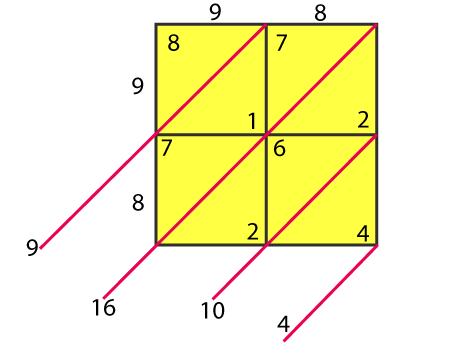∴ 982 = 9604
(ii) 273
Step 1: Obtain the number and count the number of digits in it. Let there be n digits in the number to be squared.
Step 2: Draw square and divide it into n2 sub-squares of the same size by drawing (n – 1) horizontal and (n – 1) vertical lines.
Step 3: Draw the diagonals of each sub-square.
Step 4: Write the digits of the number to be squared along left vertical side sand top horizontal side of the squares.
Step 5: Multiply each digit on the left of the square with each digit on top of the column one-by-one. Write the units digit of the product below the diagonal and tens digit above the diagonal of the corresponding sub-square.
Step 6: Starting below the lowest diagonal sum the digits along the diagonals so obtained. Write the units digit of the sum and take carry, the tens digit (if any) to the diagonal above.
Step 7: Obtain the required square by writing the digits from the left-most side.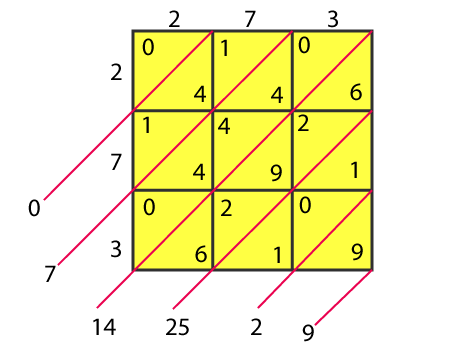∴ 2732 = 74529
(iii) 348
Step 1: Obtain the number and count the number of digits in it. Let there be n digits in the number to be squared.
Step 2: Draw square and divide it into n2 sub-squares of the same size by drawing (n – 1) horizontal and (n – 1) vertical lines.
Step 3: Draw the diagonals of each sub-square.
Step 4: Write the digits of the number to be squared along left vertical side sand top horizontal side of the squares.
Step 5: Multiply each digit on the left of the square with each digit on top of the column one-by-one. Write the units digit of the product below the diagonal and tens digit above the diagonal of the corresponding sub-square.
Step 6: Starting below the lowest diagonal sum the digits along the diagonals so obtained. Write the units digit of the sum and take carry, the tens digit (if any) to the diagonal above.
Step 7: Obtain the required square by writing the digits from the left-most side.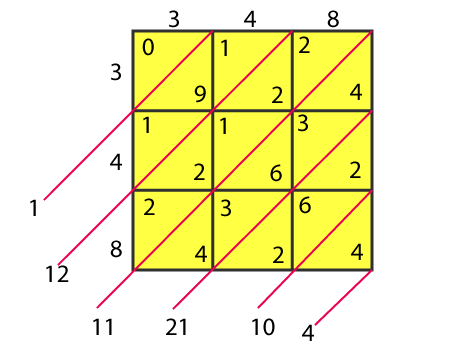∴ 3482 = 121104
(iv) 295
Step 1: Obtain the number and count the number of digits in it. Let there be n digits in the number to be squared.
Step 2: Draw square and divide it into n2 sub-squares of the same size by drawing (n – 1) horizontal and (n – 1) vertical lines.
Step 3: Draw the diagonals of each sub-square.
Step 4: Write the digits of the number to be squared along left vertical side sand top horizontal side of the squares.
Step 5: Multiply each digit on the left of the square with each digit on top of the column one-by-one. Write the units digit of the product below the diagonal and tens digit above the diagonal of the corresponding sub-square.
Step 6: Starting below the lowest diagonal sum the digits along the diagonals so obtained. Write the units digit of the sum and take carry, the tens digit (if any) to the diagonal above.
Step 7: Obtain the required square by writing the digits from the left-most side.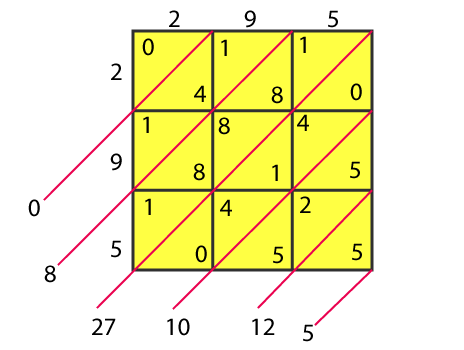∴ 2952 = 87025
(v) 171
Step 1: Obtain the number and count the number of digits in it. Let there be n digits in the number to be squared.
Step 2: Draw square and divide it into n2 sub-squares of the same size by drawing (n – 1) horizontal and (n – 1) vertical lines.
Step 3: Draw the diagonals of each sub-square.
Step 4: Write the digits of the number to be squared along left vertical side sand top horizontal side of the squares.
Step 5: Multiply each digit on the left of the square with each digit on top of the column one-by-one. Write the units digit of the product below the diagonal and tens digit above the diagonal of the corresponding sub-square.
Step 6: Starting below the lowest diagonal sum the digits along the diagonals so obtained. Write the units digit of the sum and take carry, the tens digit (if any) to the diagonal above.
Step 7: Obtain the required square by writing the digits from the left-most side.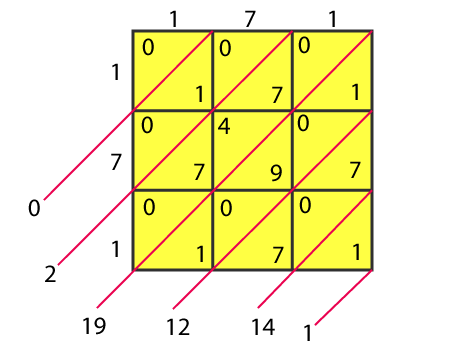∴ 1712 = 29241
3. Find the squares of the following numbers:
(i) 127
(ii) 503
(iii) 450
(iv) 862
(v) 265
Solution:
(i) 127
1272 = 127 × 127 = 16129
(ii) 503
5032 = 503 × 503 = 253009

(iii)
450
4502 = 450 × 450 = 203401
(iv) 862
8622 = 862 × 862 = 743044

(v)
265
2652 = 265 × 265 = 70225
4. Find the squares of the following numbers:
(i) 425
(ii) 575
(iii)405
(iv) 205
(v) 95
(vi) 745
(vii) 512
(viii) 995
Solution:
(i)425
4252 = 425 × 425 = 180625
(ii) 575
5752 = 575 × 575 = 330625

(iii)405
4052 = 405 × 405 = 164025
(iv) 205
2052 = 205 × 205 = 42025

(v) 95
952 = 95 × 95 = 9025
(vi) 745
7452 = 745 × 745 = 555025

(vii) 512
5122 = 512 × 512 = 262144
(viii) 995
9952 = 995 × 995 = 990025
5. Find the squares of the following numbers using the identify (a+b) 2= a2+2ab+b2:
(i) 405
(ii) 510
(iii) 1001
(iv) 209
(v) 605
Solution:
(i) 405
We know, (a+b) 2= a2+2ab+b2
405 = (400+5)2
= (400)2 + 52 + 2 (400) (5)
= 160000 + 25 + 4000
= 164025
(ii) 510
We know, (a+b) 2= a2+2ab+b2
510 = (500+10)2
= (500)2 + 102 + 2 (500) (10)
= 250000 + 100 + 10000
= 260100

(iii)
1001
We know, (a+b) 2= a2+2ab+b2
1001 = (1000+1)2
= (1000)2 + 12 + 2 (1000) (1)
= 1000000 + 1 + 2000
= 1002001

(iv)
209
We know, (a+b) 2= a2+2ab+b2
209 = (200+9)2
= (200)2 + 92 + 2 (200) (9)
= 40000 + 81 + 3600
= 43681

(v)
605
We know, (a+b) 2= a2+2ab+b2
605 = (600+5)2
= (600)2 + 52 + 2 (600) (5)
= 360000 + 25 + 6000
= 366025
6. Find the squares of the following numbers using the identity (a-b) 2= a2-2ab+b2
(i) 395
(ii) 995
(iii)495
(iv) 498
(v) 99
(vi) 999
(vii)599
Solution:
(i) 395
We know, (a-b) 2= a2-2ab+b2
395 = (400-5)2
= (400)2 + 52 – 2 (400) (5)
= 160000 + 25 – 4000
= 156025
(ii) 995
We know, (a-b) 2= a2-2ab+b2
995 = (1000-5)2
= (1000)2 + 52 – 2 (1000) (5)
= 1000000 + 25 – 10000
= 990025

(iii)
495
We know, (a-b) 2= a2-2ab+b2
495 = (500-5)2
= (500)2 + 52 – 2 (500) (5)
= 250000 + 25 – 5000
= 245025
(iv) 498
We know, (a-b) 2= a2-2ab+b2
498 = (500-2)2
= (500)2 + 22 – 2 (500) (2)
= 250000 + 4 – 2000
= 248004

(v)
99
We know, (a-b) 2= a2-2ab+b2
99 = (100-1)2
= (100)2 + 12 – 2 (100) (1)
= 10000 + 1 – 200
= 9801
(vi) 999
We know, (a-b) 2= a2-2ab+b2
999 = (1000-1)2
= (1000)2 + 12 – 2 (1000) (1)
= 1000000 + 1 – 2000
= 998001

(vii)
599
We know, (a-b) 2= a2-2ab+b2
599 = (600-1)2
= (600)2 + 12 – 2 (600) (1)
= 360000 + 1 – 1200
= 358801
7. Find the squares of the following numbers by visual method:
(i) 52
(ii) 95
(iii) 505
(iv) 702
(v) 99
Solution:
(i) 52
We know, (a+b) 2= a2+2ab+b2
52 = (50+2)2
= (50)2 + 22 + 2 (50) (2)
= 2500 + 4 + 200
= 2704
(ii) 95
We know, (a-b) 2= a2-2ab+b2
95 = (100-5)2
= (100)2 + 52 – 2 (100) (5)
= 10000 + 25 – 1000
= 9025

(iii)
505
We know, (a+b) 2= a2+2ab+b2
505 = (500+5)2
= (500)2 + 52 + 2 (500) (5)
= 250000 + 25 + 5000
= 255025
(iv) 702
We know, (a+b) 2= a2+2ab+b2
702 = (700+2)2
= (700)2 + 22 + 2 (700) (2)
= 490000 + 4 + 2800
= 492804

(v)
99
We know, (a-b) 2= a2-2ab+b2
99 = (100-1)2
= (100)2 + 12 – 2 (100) (1)
= 10000 + 1 – 200
= 9801

## EXERCISE 3.4 PAGE NO: 3.38

1.Write the possible unit’s digits of the square root of the following numbers. Which of these numbers are odd square roots?
(i) 9801
(ii) 99856
(iii) 998001
(iv) 657666025
Solution:
(i) 9801
We know that unit digit of 9801 is 1
Unit digit of square root = 1 or 9
Since the number is odd, square root is also odd
(ii) 99856
We know that unit digit of 99856 = 6
Unit digit of square root = 4 or 6
Since the number is even, square root is also even
(iii) 998001
We know that unit digit of 998001 = 10
Unit digit of square root = 10 or 9
Since the number is odd, square root is also odd
(iv) 657666025
We know that unit digit of 657666025 = 5
Unit digit of square root = 5
Since the number is odd, square root is also odd
2. Find the square root of each of the following by prime factorization.
(i) 441 (ii) 196
(iii) 529 (iv) 1764
(v) 1156 (vi) 4096
(vii) 7056 (viii) 8281
(ix) 11664 (x) 47089
(xi) 24336 (xii) 190969
(xiii) 586756 (xiv) 27225
(xv) 3013696
Solution:
(i) 441
Firstly let’s find the prime factors for
441 = 3×3×7×7
= 32×72
√441 = 3×7
= 21
(ii) 196
Firstly let’s find the prime factors for
196 = 2×2×7×7
= 22×72
√196 = 2×7
= 14

(iii)
529
Firstly let’s find the prime factors for
529 = 23×23
= 232
√529 = 23
(iv) 1764
Firstly let’s find the prime factors for
1764 = 2×2×3×3×7×7
= 22×32×72
√1764 = 2×3×7
= 42

(v)
1156
Firstly let’s find the prime factors for
1156 = 2×2×17×17
= 22×172
√1156 = 2×17
= 34
(vi) 4096
Firstly let’s find the prime factors for
4096 = 2×2×2×2×2×2×2×2×2×2×2×2
= 212
√4096 = 26
= 64
(vii) 7056
Firstly let’s find the prime factors for
7056 = 2×2×2×2×21×21
= 22×22×212
√7056 = 2×2×21
= 84
(viii) 8281
Firstly let’s find the prime factors for
8281 = 91×91
= 912
√8281 = 91

(ix)
11664
Firstly let’s find the prime factors for
11664 = 2×2×2×2×3×3×3×3×3×3
= 22×22×32×32×32
√11664 = 2×2×3×3×3
= 108
(x) 47089
Firstly let’s find the prime factors for
47089 = 217×217
= 2172
√47089 = 217

(xi)
24336
Firstly let’s find the prime factors for
24336 = 2×2×2×2×3×3×13×13
= 22×22×32×132
√24336 = 2×2×3×13
= 156
(xii) 190969
Firstly let’s find the prime factors for
190969 = 23×23×19×19
= 232×192
√190969 = 23×19
= 437

(xiii)
586756
Firstly let’s find the prime factors for
586756 = 2×2×383×383
= 22×3832
√586756 = 2×383
= 766
(xiv) 27225
Firstly let’s find the prime factors for
27225 = 5×5×3×3×11×11
= 52×32×112
√27225 = 5×3×11
= 165

(xv)
3013696
Firstly let’s find the prime factors for
3013696 = 2×2×2×2×2×2×217×217
= 26×2172
√3013696 = 23×217
= 1736
3.Find the smallest number by which 180 must be multiplied so that it becomes a perfect square. Also, find the square root of the perfect square so obtained.
Solution:
Firstly let’s find the prime factors for
180 = (2 × 2) × (3 × 3) × 5
=22 × 32 × 5
To make the unpaired 5 into paired, multiply the number with 5
180 × 5 = 22 × 32 × 52
∴ Square root of √ (180 × 5) = 2 × 3 × 5
= 30
4. Find the smallest number by which 147 must be multiplied so that it becomes a perfect square. Also, find the square root of the number so obtained.
Solution:
Firstly let’s find the prime factors for
147 = (7 × 7) × 3
=72 × 3
To make the unpaired 3 into paired, multiply the number with 3
147 × 3 = 72 × 32
∴ Square root of √ (147 × 3) = 7 × 3
= 21
5. Find the smallest number by which 3645 must be divided so that it becomes a perfect square. Also, find the square root of the resulting number.
Solution:
Firstly let’s find the prime factors for
3645 = (3 × 3) × (3 × 3) × 5 × 3
=32 × 32 × 5 × 3
To make the unpaired 5 and 3 into paired, the number 3645 has to be divided by 5 × 3=15
3645 ÷ 15 = 32 × 32
∴ Square root of √ (3645 ÷ 15) = 3 × 3
= 9
6. Find the smallest number by which 1152 must be divided so that it becomes a square. Also, find the square root of the number so obtained.
Solution:
Firstly let’s find the prime factors for
1152 = (2 × 2) × (2 × 2) × (2 × 2) × 2 × (3 × 3)
=22 × 22 × 22 × 32 × 2
To make the unpaired 2 into paired, the number 1152 has to be divided by 2
1152 ÷ 2 = 22 × 2× 22 × 32
∴ Square root of √ (1152 ÷ 2) = 2 × 2 × 2 × 3
= 24
7. The product of two numbers is 1296. If one number is 16 times the other, find the numbers.
Solution:
Let us consider two numbers a and b
So we know that one of the number, a =16b
a × b = 1296
16b × b = 1296
16b2 = 1296
b2 = 1296/16 = 81
b = 9
a = 16b
= 16(9)
= 144
∴ a =144 and b =9
8. A welfare association collected Rs 202500 as donation from the residents. If each paid as many rupees as there were residents, find the number of residents.
Solution:
Let us consider total residents as a
So, each paid Rs. a
Total collection = a (a) = a2
We know that the total Collection = 202500
a = √ 202500
a = √a(2 × 2 × 3 × 3 × 3 × 3 × 5 × 5 × 5 × 5)
= 2 × 3 × 3 × 5 × 5a
= 450
∴ Total residents = 450
9. A society collected Rs 92.16. Each member collected as many paise as there were members. How many members were there and how much did each contribute?
Solution:
Let us consider there were few members, each attributed a paise
a (a), i.e. total cost collected = 9216 paise
a2 = 9216
a = √9216
= 2 × 2 × 2 × 12
= 96
∴ There were 96 members in the society and each contributed 96 paise
10. A society collected Rs 2304 as fees from its students. If each student paid as many paise as there were students in the school, how many students were there in the school?
Solution:
Let us consider number of school students as a
each student contributed a paise
Total money obtained = a2paise
= 230400 paise
a = √230400
= √2304 × √100
= 10√2304
a = 10 × 2 × 2 × 12
a = 480
∴ There were 480 students in the school
11. The area of a square field is 5184 m2. A rectangular field, whose length is twice its breadth has its perimeter equal to the perimeter of the square field. Find the area of the rectangular field.
Solution:
Let us consider the side of square field as a
a2 = 5184 m2
a = √5184m
a = 2 × 2 ×2 × 9
= 72 m
Perimeter of square = 4a
= 4(72)
= 288 m
Perimeter of rectangle = 2 (l + b) = perimeter of the square field
= 288 m
2 (2b + b) = 288
b = 48 and l = 96
Area of rectangle = 96 × 48 m2
= 4608 m2
12. Find the least square number, exactly divisible by each one of the numbers: (i) 6, 9, 15 and 20 (ii) 8, 12, 15 and 20
Solution:
(i) 6, 9, 15 and 20
Firstly take L.C.M for 6, 9, 15, 20 which is 180
So the prime factors of 180 = 22 × 32 × 5
To make it a perfect square, we have to multiply the number with 5
180 × 5 = 22 × 32 × 52
∴ 900 is the least square number divisible by 6, 9, 15 and 20
(ii) 8, 2, 15 and 20
Firstly take L.C.M for 8, 2, 15, 20 which is 360
So the prime factors of 360 = 22 × 32 ×2 × 5
To make it a perfect square, we have to multiply the number with 2 × 5 = 10
360 × 10 = 22 × 32 × 52 × 22
∴ 3600 is the least square number divisible by 8, 12, 15 and 20
13. Find the square roots of 121 and 169 by the method of repeated subtraction.
Solution:
Let us find the square roots of 121 and 169 by the method of repeated subtraction
121 – 1 = 120
120 – 3 = 117
117 – 5 = 112
112 – 7 = 115
115 – 9 = 106
106 – 11 = 95
95 – 13 = 82
82 – 15 = 67
67 – 17 = 50
50 – 19 = 31
31 – 21 = 10
Clearly, we have performed operation 11 times
∴ √121 = 11
169 – 1 = 168
168 – 3 = 165
165 – 5 = 160
160 – 7 = 153
153 – 9 = 144
144 – 11 = 133
133 – 13 = 120
120 – 15 = 105
105 – 17 = 88
88 – 19 = 69
69 – 21 = 48
48 – 23 = 25
25 – 25 = 0
Clearly, we have performed subtraction 13 times
∴ √169 = 13
14. Write the prime factorization of the following numbers and hence find their square roots.
(i) 7744
(ii) 9604
(iii) 5929
(iv) 7056
Solution:
(i) 7744
Prime factors of 7744 is
7744 = 22 × 22 × 22 × 112
∴ The square root of 7744 is
√7744 = 2 × 2 × 2 × 11
= 88
(ii) 9604
Prime factors of 9604 is
9604 = 22 × 72 × 72
∴ The square root of 9604 is
√9604 = 2 × 7 × 7
= 98
(iii) 5929
Prime factors of 5929 is
5929 = 112 × 72
∴ The square root of 5929 is
√5929 = 11 × 7
= 77
(iv) 7056
Prime factors of 7056 is
7056 = 22 × 22 × 72 × 32
∴ The square root of 7056 is
√7056 = 2 × 2 × 7 × 3
= 84
15. The students of class VIII of a school donated Rs 2401 for PM’s National Relief Fund. Each student donated as many rupees as the number of students in the class, Find the number of students in the class.
Solution:
Let us consider number of students as a
Each student denoted a rupee
So, total amount collected is a × a rupees = 2401
a2 = 2401
a = √2401
a = 49
∴ There are 49 students in the class.
16. A PT teacher wants to arrange maximum possible number of 6000 students in a field such that the number of rows is equal to the number of columns. Find the number of rows if 71 were left out after arrangement.
Solution:
Let us consider number of rows as a
No. of columns = a
Total number of students who sat in the field = a2
Total students a2 + 71 = 6000
a2 = 5929
a = √5929
a = 77
∴ total number of rows are 77.

## EXERCISE 3.5 PAGE NO: 3.43

1.Find the square root of each of the following by long division method:
(i) 12544 (ii) 97344
(iii) 286225 (iv) 390625
(v) 363609 (vi) 974169
(vii) 120409 (viii) 1471369
(ix) 291600 (x) 9653449
(xi) 1745041 (xii) 4008004
(xiii) 20657025 (xiv) 152547201
(xv) 20421361 (xvi)62504836
(xvii) 82264900 (xviii) 3226694416
(xix) 6407522209 (xx) 3915380329
Solution:
(i) 12544
By using long division method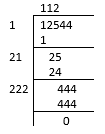∴ the square root of 12544
√12544 = 112
(ii) 97344
By using long division method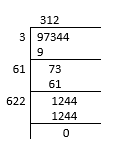∴ the square root of 97344
√97344 = 312

(iii)
286225
By using long division method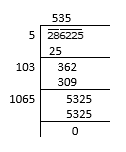∴ the square root of 286225
√286225 = 535
(iv) 390625
By using long division method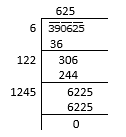∴ the square root of 390625
√390625 = 625

(v)
363609
By using long division method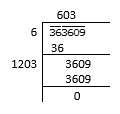∴ the square root of 363609
√36369 = 603
(vi) 974169
By using long division method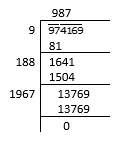∴ the square root of 974169
√974169 = 987
(vii) 120409
By using long division method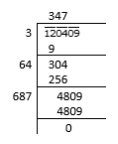∴ the square root of 120409
√120409 = 347
(viii) 1471369
By using long division method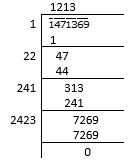∴ the square root of 1471369
√1471369 = 1213

(ix)
291600
By using long division method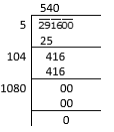∴ the square root of 291600
√291600 = 540
(x) 9653449
By using long division method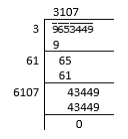∴ the square root of 9653449
√9653449 = 3107

(xi)
1745041
By using long division method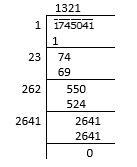∴ the square root of 1745041
√1745041 = 1321
(xii) 4008004
By using long division method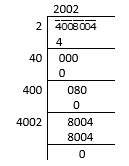∴ the square root of 4008004
√4008004 = 2002

(xiii)
20657025
By using long division method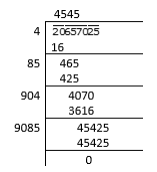∴ the square root of 20657025
√20657025 = 4545
(xiv) 152547201
By using long division method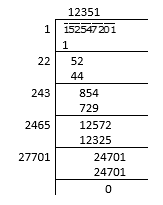∴ the square root of 152547201
√152547201 = 12351

(xv)
20421361
By using long division method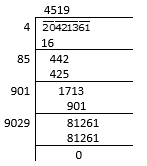∴ the square root of 20421361
√20421361 = 4519
(xvi) 62504836
By using long division method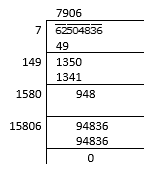∴ the square root of 62504836
√62504836 = 7906

(xvii)
82264900
By using long division method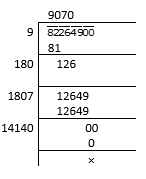∴ the square root of 82264900
√82264900 = 9070
(xviii) 3226694416
By using long division method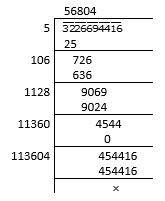∴ the square root of 3226694416
√3226694416 = 56804

(xix)
6407522209
By using long division method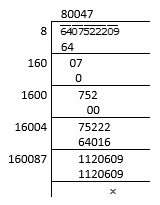∴ the square root of 6407522209
√6407522209 = 80047
(xx) 3915380329
By using long division method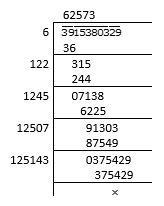∴ the square root of 3915380329
√3915380329 = 62573
2. Find the least number which must be subtracted from the following numbers to make them a perfect square:
(i) 2361
(ii) 194491
(iii) 26535
(iv) 161605
(v) 4401624
Solution:
(i) 2361
By using long division method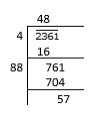∴ 57 has to be subtracted from 2361 to get a perfect square.

(ii)
194491
By using long division method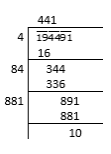∴ 10 has to be subtracted from 194491 to get a perfect square.

(iii)
26535
By using long division method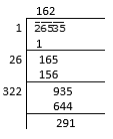∴ 291 has to be subtracted from 26535 to get a perfect square.

(iv)
161605
By using long division method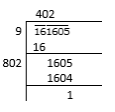∴ 1 has to be subtracted from 161605 to get a perfect square.

(v)
4401624
By using long division method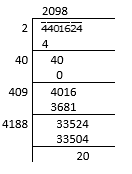∴ 20 has to be subtracted from 4401624 to get a perfect square.
3. Find the least number which must be added to the following numbers to make them a perfect square:
(i) 5607
(ii)4931
(iii) 4515600
(iv) 37460
(v) 506900
Solution:
(i) 5607
By using long division method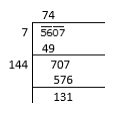The remainder is 131
Since, (74)2 < 5607
We take, the next perfect square number i.e., (75)2
(75)2 = 5625 > 5607
So, the number to be added = 5625 – 5607 = 18

(ii)
4931
By using long division method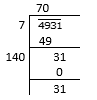The remainder is 31
Since, (70)2 < 4931
We take, the next perfect square number i.e., (71)2
(71)2 = 5041 > 4931
So, the number to be added = 5041 – 4931 = 110

(iii)
4515600
By using long division method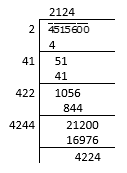The remainder is 4224
Since, (2124)2 < 4515600
We take, the next perfect square number i.e., (2125)2
(2125)2 = 4515625 > 4515600
So, the number to be added = 4515625 – 4515600 = 25

(iv)
37460
By using long division method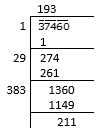The remainder is 211
Since, (193)2 < 37460
We take, the next perfect square number i.e., (194)2
(194)2 = 37636 > 37460
So, the number to be added = 37636 – 37460 = 176

(v)
506900
By using long division method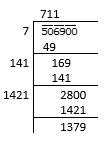The remainder is 1379
Since, (711)2 < 506900
We take, the next perfect square number i.e., (712)2
(712)2 = 506944 > 506900
So, the number to be added = 506944 – 506900 = 44
4. Find the greatest number of 5 digits which is a perfect square.
Solution:
We know that the greatest 5 digit number is 99999
By using long division method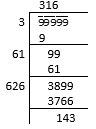The remainder is 143
So, the greatest 5 digit perfect square number is:
99999 – 143 = 99856
∴ 99856 is the required greatest 5 digit perfect square number.
5. Find the least number of 4 digits which is a perfect square.
Solution:
We know that the least 4 digit number is 1000
By using long division method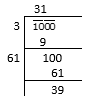The remainder is 39
Since, (31)2 < 1000
We take, the next perfect square number i.e., (32)2
(32)2 = 1024 > 1000
∴ 1024 is the required least number 4 digit number which is a perfect square.
6. Find the least number of six digits which is a perfect square.
Solution:
We know that the least 6 digit number is 100000
By using long division method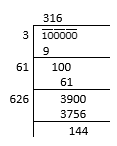The remainder is 144
Since, (316)2 < 100000
We take, the next perfect square number i.e., (317)2
(317)2 = 100489 > 100000
∴ 100489 is the required least number 6 digit number which is a perfect square.
7. Find the greatest number of 4 digits which is a perfect square.
Solution:
We know that the greatest 4 digit number is 9999
By using long division method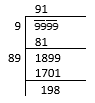The remainder is 198
So, the greatest 4 digit perfect square number is:
9999 – 198 = 9801
∴ 9801 is the required greatest 4 digit perfect square number.
8. A General arranges his soldiers in rows to form a perfect square. He finds that in doing so, 60 soldiers are left out. If the total number of soldiers be 8160, find the number of soldiers in each row
Solution:
We know that the total number of soldiers = 8160
Number of soldiers left out = 60
Number of soldiers arranged in rows to form a perfect square = 8160 – 60 = 8100
∴ number of soldiers in each row = √8100
= √ (9×9×10×10)
= 9×10
= 90
9. The area of a square field is 60025m2. A man cycles along its boundary at 18 Km/hr. In how much time will he return at the starting point?
Solution:
We know that the area of square field = 60025 m2
Speed of cyclist = 18 km/h
= 18 × (1000/60×60)
= 5 m/s2
Area = 60025 m2
Side2 = 60025
Side = √60025
= 245
We know, Total length of boundary = 4 × Side
= 4 × 245
= 980 m
= 196 seconds
= 3 minutes 16 seconds
10. The cost of levelling and turning a square lawn at Rs 2.50 per m2 is Rs13322.50 Find the cost of fencing it at Rs 5 per metre.
Solution:
We know that the cost of levelling and turning a square lawn = 2.50 per m2
Total cost of levelling and turning = Rs. 13322.50
Total area of square lawn = 13322.50/2.50
= 5329 m2
Side2 = 5329
Side of square lawn = √5329
= 73 m
So, total length of lawn = 4 × 73
= 292 m
∴ Cost of fencing the lawn at Rs 5 per metre = 292 × 5
= Rs. 1460
11. Find the greatest number of three digits which is a perfect square.
Solution:
We know that the greatest 3 digit number is 999
By using long division method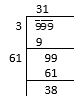The remainder is 38
So, the greatest 3 digit perfect square number is:
999 – 38 = 961
∴ 961 is the required greatest 3 digit perfect square number.
12. Find the smallest number which must be added to 2300 so that it becomes a perfect square.
Solution:
By using long division method let’s find the square root of 2300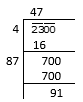The remainder is 91
Since, (47)2 < 2300
We take, the next perfect square number i.e., (48)2
(48)2 = 2304 > 2300
∴ The smallest number required to be added to 2300 to get a perfect square is
2304 – 2300 = 4

## EXERCISE 3.6 PAGE NO: 3.48

1. Find the square root of:
(i) 441/961
(ii) 324/841
(iii) 4 29/29
(iv) 2 14/25
(v) 2 137/196
(vi) 23 26/121
(vii) 25 544/729
(viii) 75 46/49
(ix) 3 942/2209
(x) 3 334/3025
(xi) 21 2797/3364
(xii) 38 11/25
(xiii) 23 394/729
(xiv) 21 51/169
(xv) 10 151/225
Solution:
(i) 441/961
The square root of
√441/961 = 21/31
(ii) 324/841
The square root of
√324/841= 18/29
(iii) 4 29/29
The square root of
√(4 29/29) = √(225/49) = 15/7
(iv) 2 14/25
The square root of
√(2 14/25) = √(64/25) = 8/5
(v) 2 137/196
The square root of
√2 137/196 = √ (529/196) = 23/14
(vi) 23 26/121
The square root of
√(23 26/121) = √(2809/121) = 53/11
(vii) 25 544/729
The square root of
√(25 544/729) = √(18769/729) = 137/27
(viii) 75 46/49
The square root of
√(75 46/49) = √(3721/49) = 61/7
(ix) 3 942/2209
The square root of
√(3 942/2209) = √(7569/2209) = 87/47
(x) 3 334/3025
The square root of
√(3 334/3025) = √(9409/3025) = 97/55
(xi) 21 2797/3364
The square root of
√(21 2797/3364) = √(73441/3364) = 271/58
(xii) 38 11/25
The square root of
√(38 11/25) = √(961/25) = 31/5
(xiii) 23 394/729
The square root of
√(23 394/729) = √(17161/729) = 131/27 = 4 23/27
(xiv) 21 51/169
The square root of
√(21 51/169) = √(3600/169) = 60/13 = 4 8/13
(xv) 10 151/225
The square root of
√(10 151/225) = √(2401/225) = 49/15 = 3 4/15
2. Find the value of:
(i) √80/√405
(ii) √441/√625
(iii) √1587/√1728
(iv) √72 ×√338
(v) √45 × √20
Solution:
(i) √80/√405
√80/√405 = √16/√81 = 4/9
(ii) √441/√625
√441/√625 = 21/25
(iii) √1587/√1728
√1587/√1728 = √529/√576 = 23/24
(iv) √72 ×√338
√72 ×√338 = √(2×2×2×3×3) ×√(2×13×13)
By using the formula √a × √b = √(a×b)
= √(2×2×2×3×3×2×13×13)
= 22 × 3 × 13
= 156
(v) √45 × √20
√45 × √20 = √(5×9×9) × √(5×2×2)
By using the formula √a × √b = √(a×b)
= √(5×9×9×5×2×2)
= 5 × 9 × 2
= 90
3. The area of a square field is 80 244/729 square metres. Find the length of each side of the field.
Solution:
We know that the given area = 80 244/729 m2
= 58564/729 m2
If L is length of each side
L2 = 58564/729
L = √ (58564/729) = √58564/√729
= 242/27
= 8 26/27
∴ Length is 8 26/27
4. The area of a square field is 30 1/4m2. Calculate the length of the side of the square.
Solution:
We know that the given area = 30 1/4 m2
= 121/4 m2
If L is length of each side then,
L2 = 121/4
L = √(121/4)  = √121/√4
= 11/2
∴ Length is 11/2
5. Find the length of a side of a square playground whose area is equal to the area of a rectangular field of dimensions 72m and 338 m.
Solution:
By using the formula
Area of rectangular field = l × b
= 72 × 338 m2
= 24336 m2
Area of square, L2 = 24336 m2
L = √24336
= 156 m
∴ Length of side of square playground is 156 m.

## EXERCISE 3.7 PAGE NO: 3.52

Find the square root of the following numbers in decimal form:
1. 84.8241
Solution:
By using long division method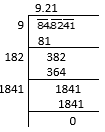∴ the square root of 84.8241
√84.8241 = 9.21
2. 0.7225
Solution:
By using long division method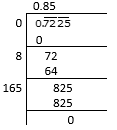∴ the square root of 0.7225
√0.7225 = 0.85
3. 0.813604
Solution:
By using long division method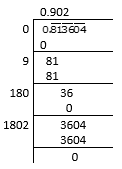∴ the square root of 0.813604
√0.813604 = 0.902
4. 0.00002025
Solution:
By using long division method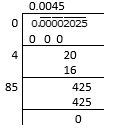∴ the square root of 0.00002025
√0.00002025 = 0.0045
5. 150.0625
Solution:
By using long division method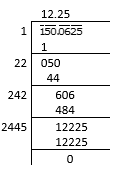∴ the square root of 150.0625
√150.0625 = 12.25
6. 225.6004
Solution:
By using long division method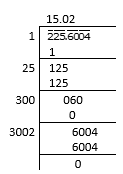∴ the square root of 225.6004
√225.6004 = 15.02
7. 3600.720036
Solution:
By using long division method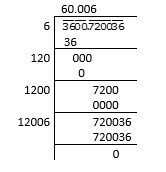∴ the square root of 3600.720036
√3600.720036 = 60.006
8. 236.144689
Solution:
By using long division method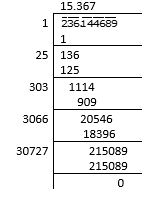∴ the square root of 236.144689
√236.144689 = 15.367
9. 0.00059049
Solution:
By using long division method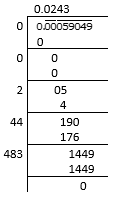∴ the square root of 0.00059049
√0.00059049 = 0.0243
10. 176.252176
Solution:
By using long division method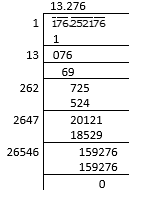∴ the square root of 176.252176
√176.252176 = 13.276
11. 9998.0001
Solution:
By using long division method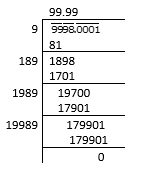∴ the square root of 9998.0001
√9998.0001 = 99.99
12. 0.00038809
Solution:
By using long division method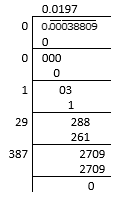∴ the square root of 0.00038809
√0.00038809 = 0.0197
13. What is that fraction which when multiplied by itself gives 227.798649?
Solution:
Let us consider a number a
Where, a = √227.798649
= 15.093
By using long division method let us verify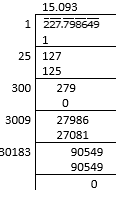∴ 15.093 is the fraction which when multiplied by itself gives 227.798649.
14. The area of a square playground is 256.6404 square meter. Find the length of one side of the playground.
Solution:
We know that the given area of a square playground = 256.6404
i.e., L2 = 256.6404 m2
L = √256.6404
= 16.02m
By using long division method let us verify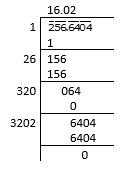∴ length of one side of the playground is 16.02m.
15. What is the fraction which when multiplied by itself gives 0.00053361?
Solution:
Let us consider a number a
Where, a = √0.00053361
= 0.0231
By using long division method let us verify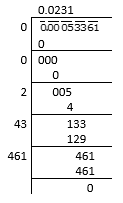∴ 0.0231 is the fraction which when multiplied by itself gives 0.00053361.
16. Simplify:
(i) (√59.29 – √5.29)/ (√59.29 + √5.29)
(ii) (√0.2304 + √0.1764)/ (√0.2304 – √0.1764)
Solution:
(i) (√59.29 – √5.29)/ (√59.29 + √5.29)
Firstly let us find the square root √59.29 and √5.29
√59.29 = √5929/ √100
= 77/10
= 7.7
√5.29 = √5.29/ √100
= 23/10
= 2.3
So, (7.7 – 2.3)/ (7.7 + 2.3)
= 54/10
= 0.54
(ii) (√0.2304 + √0.1764)/ (√0.2304 – √0.1764)
Firstly let us find the square root √0.2304 and √0.1764
√0.2304 = √2304/ √10000
= 48/100
= 0.48
√0.1764 = √1764/ √10000
= 42/100
= 0.42
So, (0.48 + 0.42)/ (0.48 – 0.42)
= 0.9/0.06
= 15
17. Evaluate √50625 and hence find the value of √506.25 + √5.0625
Solution:
By using long division method let us find the √50625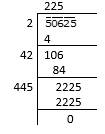So now, 506.25 = √50625/ √100
= 225/10
= 22.5
√5.0625 = √50625/ √10000
= 225/100
= 2.25
So equating in the above equation we get,
√506.25 + √5.0625 = 22.5 + 2.25
= 24.75
18. Find the value of √103.0225 and hence find the value of
(i) √10302.25
(ii) √1.030225
Solution:
By using long division method let us find the √103.0225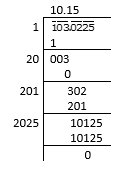So now, (i)10302.25 = √(10302.25× 100)
= 10 × 10.15
= 101.5
(ii)√1.030225 = √1.030225/ √1000
= 10.15/10
= 1.015

## EXERCISE 3.8 PAGE NO: 3.56

1. Find the square root of each of the following correct to three places of decimal.
(i) 5 (ii) 7
(iii) 17 (iv) 20
(v) 66 (vi) 427
(vii) 1.7 (viii) 23.1
(ix) 2.5 (x) 237.615
(xi) 15.3215 (xii) 0.9
(xiii) 0.1 (xiv) 0.016
(xv) 0.00064 (xvi) 0.019
(xvii) 7/8 (xviii) 5/12
(xix) 2 1/2 (xx) 287 5/8
Solution:
(i) 5
By using long division method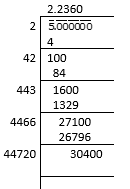∴ the square root of 5 is 2.236
(ii) 7
By using long division method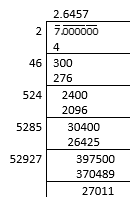∴ the square root of 7 is 2.646

(iii)
17
By using long division method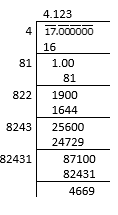∴ the square root of 17 is 4.123
(iv) 20
By using long division method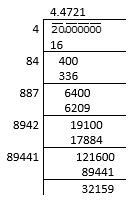∴ the square root of 20 is 4.472

(v)
66
By using long division method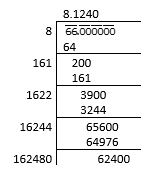∴ the square root of 66 is 8.124
(vi) 427
By using long division method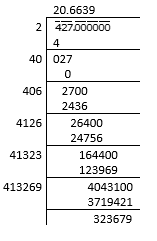∴ the square root of 427 is 20.664

(vii)
1.7
By using long division method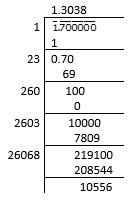∴ the square root of 1.7 is 1.304
(viii) 23.1
By using long division method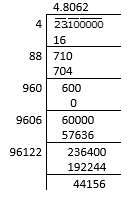∴ the square root of 23.1 is 4.806

(ix)
2.5
By using long division method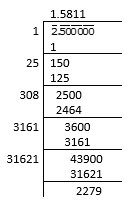∴ the square root of 2.5 is 1.581
(x) 237.615
By using long division method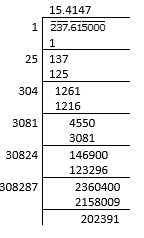∴ the square root of 237.615 is 15.415

(xi)
15.3215
By using long division method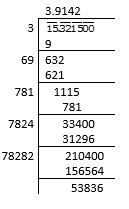∴ the square root of 15.3215 is 3.914
(xii) 0.9
By using long division method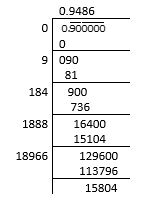∴ the square root of 0.9 is 0.949

(xiii)
0.1
By using long division method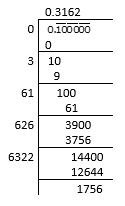∴ the square root of 0.1 is 0.316
(xiv) 0.016
By using long division method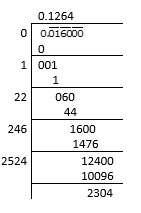∴ the square root of 0.016 is 0.126

(xv)
0.00064
By using long division method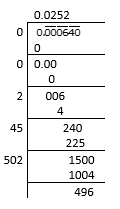∴ the square root of 0.00064 is 0.025
(xvi) 0.019
By using long division method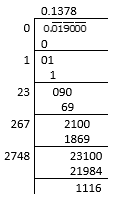∴ the square root of 0.019 is 0.138

(xvii)
7/8
By using long division method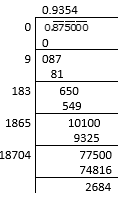∴ the square root of 7/8 is 0.935
(xviii) 5/12
By using long division method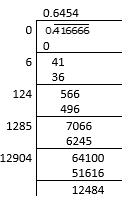∴ the square root of 5/12 is 0.645

(xix)
2 1/2
By using long division method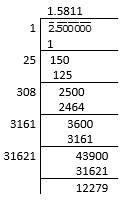∴ the square root of 5/2 is 1.581
(xx) 287 5/8
By using long division method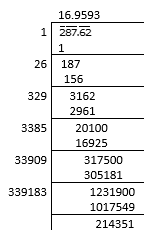∴ the square root of 2301/8 is 16.960
2. Find the square root of 12.0068 correct to four decimal places.
Solution:
By using long division method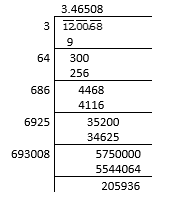∴ the square root of 12.0068 is 3.4651
3. Find the square root of 11 correct to five decimal places.
Solution:
By using long division method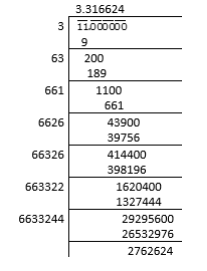∴ the square root of 11 is 3.31662
4. Give that: √2 = 1.414, √3 = 1.732, √5 = 2.236 and √7 = 2.646, evaluate each of the following:
(i) √ (144/7)
(ii) √ (2500/3)
Solution:
(i) √ (144/7)
Now let us simplify the given equation
√ (144/7) = √ (12×12)/ √7
= 12/ 2.646
= 4.535
(ii) √ (2500/3)
Now let us simplify the given equation
√ (2500/3) = √ (5×5×10×10) /√3
= 5×10/ 1.732
= 50/1.732
= 28.867
5. Given that √2 = 1.414, √3 = 1.732, √5 = 2.236 and √7 = 2.646 find the square roots of the following:
(i) 196/75
(ii) 400/63
(iii) 150/7
(iv) 256/5
(v) 27/50
Solution:
(i) 196/75
Let us find the square root for196/75
(196/75) = (196)/ √ (75)
(14×14)/ √ (5×5×3)
= 14/ (53)
= 14/ (5×1.732)
= 14/8.66
= 1.617

(ii)
400/63
Let us find the square root for400/63
(400/63) = (400)/ √ (63)
(20×20)/ √ (3×3×7)
= 20/ (37)
= 20/ (3×2.646)
= 20/7.938
= 2.520
(iii) 150/7
Let us find the square root for150/7
(150/7) = (150)/ √ (7)
(3×5×5×2)/ √ (7)
= (52)/ (7)
= 5×1.732×1.414/ (2.646)
= 12.245/2.646
= 4.628
(iv) 256/5
Let us find the square root for256/5
(256/5) = (256)/ √ (5)
(16×16)/ √ (5)
= 16/ (5)
= 16/2.236
= 7.155

(v)
27/50
Let us find the square root for27/50
(27/50) = (27)/ √ (50)
(3×3×3)/ √ (5×5×2)
= (33)/ (52)
= (3×1.732)/ (5×1.414)
= 5.196/7.07
= 0.735

## EXERCISE 3.9 PAGE NO: 3.61

Using square root table, find the square roots of the following:
1. 7
Solution:
From square root table we know,
Square root of 7 is:
√7 = 2.645
∴ The square root of 7 is 2.645
2. 15
Solution:
From square root table we know,
Square root of 7 is:
15 = 3.8729
∴ The square root of 15 is 3.873
3. 74
Solution:
From square root table we know,
Square root of 74 is:
74 = 8.6023
∴ The square root of 74 is 8.602
4. 82
Solution:
From square root table we know,
Square root of 82 is:
82 = 9.0553
∴ The square root of 82 is 9.055
5. 198
Solution:
From square root table we know,
Square root of 198 is:
198 = 14.0712
∴ The square root of 198 is 14.071
6. 540
Solution:
From square root table we know,
Square root of 540 is:
540 = 23.2379
∴ The square root of 540 is 23.24
7. 8700
Solution:
From square root table we know,
Square root of 8700 is:
8700 = 93.2737
∴ The square root of 8700 is 93.27
8. 3509
Solution:
From square root table we know,
Square root of 3509 is:
3509 = 59.2368
∴ The square root of 3509 is 59.235
9. 6929
Solution:
From square root table we know,
Square root of 6929 is:
6929 = 83.2406
∴ The square root of 6929 is 83.239
10. 25725
Solution:
From square root table we know,
Square root of 25725 is:
25725 = 160.3901
∴ The square root of 25725 is 160.41
11. 1312.
Solution:
From square root table we know,
Square root of 1312 is:
1312 = 36.2215
∴ The square root of 1312 is 36.22
12. 4192
Solution:
From square root table we know,
Square root of 4192 is:
4192 = 64.7456
∴ The square root of 4192 is 64.75
13. 4955
Solution:
From square root table we know,
Square root of 4955 is:
4955 = 70.3917
∴ The square root of 4955 is 70.39
14. 99/144
Solution:
From square root table we know,
Square root of 99/144 is:
(99/144) = 0.82915
∴ The square root of 99/144 is 0.829
15. 57/169
Solution:
From square root table we know,
Square root of 57/169 is:
√(57/169) = 0.58207
∴ The square root of 57/169 is 0.581
16. 101/169
Solution:
From square root table we know,
Square root of 101/169 is:
√(101/169) = 0.77306
∴ The square root of 57/169 is 0.773
17. 13.21
Solution:
From square root table we know,
Square root of 13.21 is:
√13.21 = 3.6345
∴ The square root of 13.21 is 3.635
18. 21.97
Solution:
From square root table we know,
Square root of 21.97 is:
√21.97 = 4.6872
∴ The square root of 21.97 is 4.6872
19. 110
Solution:
From square root table we know,
Square root of 110 is:
√110 = 10.4880
∴ The square root of 110 is 10.488
20. 1110
Solution:
From square root table we know,
Square root of 1110 is:
√1110 = 33.3166
∴ The square root of 1110 is 33.317
21. 11.11
Solution:
From square root table we know,
Square root of 11.11 is:
√11.11 = 3.33316
∴ The square root of 11.11 is 3.333
22. The area of a square field is 325m2. Find the approximate length of one side of the field.
Solution:
We know that the given area of the field = 325 m2
To find the approximate length of the side of the field we will have to calculate the square root of 325
√325 = 18.027 m
∴ The approximate length of one side of the field is 18.027 m
23. Find the length of a side of a square, whose area is equal to the area of a rectangle with sides 240 m and 70 m.
Solution:
We know that from the question,
Area of square = Area of rectangle
Side2 = 240 × 70
Side = √(240 × 70)
= √(10×10×2×2×2×3×7)
= 20√(42)
= 20 × 6.48
= 129.60 m
∴ The length of side of the square is 129.60 m
Courtesy : CBSE